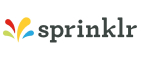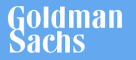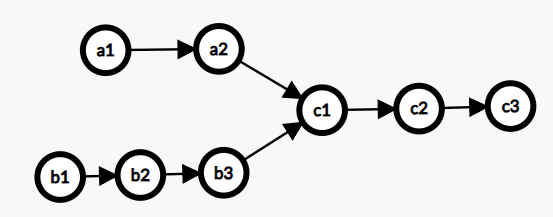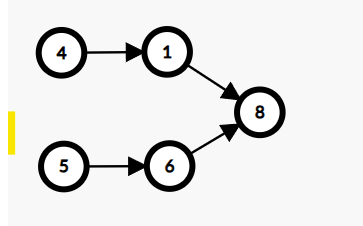New update is available. Click here to update.
Topics

# Intersection of Two Linked Lists

Easy0/40
Average time to solve is 25m+71 more companies

## Problem statement

You are given two Singly Linked Lists of integers, which may have an intersection point.

Your task is to return the first intersection node. If there is no intersection, return NULL.

Example:-
``````The Linked Lists, where a1, a2, c1, c2, c3 is the first linked list and b1, b2, b3, c1, c2, c3 is the second linked list, merging at node c1.
``````Detailed explanation ( Input/output format, Notes, Images )
Sample Input 1 :
``````4 1 -1
5 6 -1
8 -1
``````
Sample Output 1 :
``````8
``````
Explanation For Sample Input 1:
``````As shown in the diagram, the node with data is 8, at which merging starts
``````Sample Input 2 :
``````1 9 1 -1
3 -1
2 -1
``````
Sample Output 2 :
``````2
``````
Constraints :
``````-10^9 <= data <= 10^9 and data != -1
The length of both the linked list is positive.
Time Limit: 1 sec
``````Console